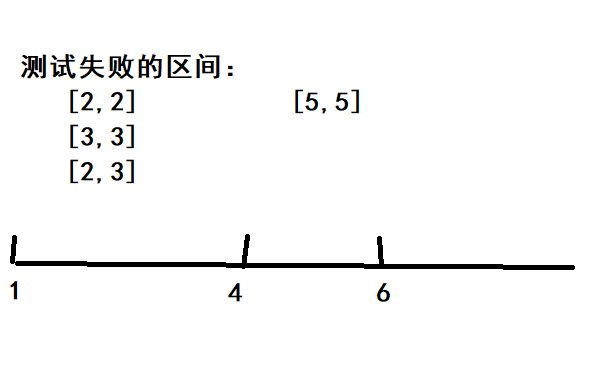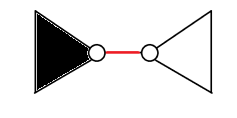AK-dream 2020-08-06T12:38:54.496Z http://akdream.tk/ AK-dream Hexo 长跑[BZOJ2959] http://akdream.tk/post/f9901713.html/ 2020-08-06T12:38:27.000Z 2020-08-06T12:38:54.496Z 题目描述

1. 修建了一条连接A地点和B地点的跑道。
2. A点的刷卡机台数变为了B。
3. 进行了一次长跑。问一个同学从A出发，最后到达B最多可以刷卡多少次。具体的要求如下：

### 题解

LCT维护边双连通分量

1. LCT内部维护一个并查集记录两个点是否在同一个边双内，外部还要维护一个并查集记录两个点当前是否联通

2. access时跳父亲要跳到find(fa[x])上，更改重儿子时还要记得把新的重儿子的父亲设为自己！

3. 如果在两点之间连边时，两点已在同一棵树中，但不在同一个边双中，则要把两点之间的路径上的点合并为一个点：

4. 进行任何操作时(例如link,split)，都是对find(x)进行修改，而不是$x$！

### 代码

]]>

• L u v w：添加一条连接 $u$ 和 $v$，长度为 $w$ 的边。
• C u v：删除连接 $u$ 和 $v$ 的边（保证存在）。
• F u：反转点 $u$ 的颜色（黑变白，白变黑）。
• Q u：询问所有与 $u$ 相连的黑点到 $u$ 的距离之和。（相连指的是在同一连通块中）

### 题解

LCT维护子树信息]]>
<h3 id="题目描述"><a href="#题目描述" class="headerlink" title="题目描述"></a>题目描述</h3><p>给你一个有 $n$ 个点的森林，点有黑白两种颜色，初始时所有点都是白色，森林的每条边有边权，初始时这个森林有 $m$ 条边。

[ZJOI2018]历史

### 题解]]>

### 输出格式

$M$ 个数表示答案。

### 题解]]>
<h3 id="题目描述"><a href="#题目描述" class="headerlink" title="题目描述"></a>题目描述</h3><p>有 $N$ 个字符串，每个字符串有一个权值 $v_i$。随后给出 $M$ 次询问，每次对一个区间进行检测。令最长的字符串长度

JOI 王国共有 $N$ 个城市，这些城市从 $1$ 到 $N$ 编号。共有 $M$ 条公交线路连接这些城市，这些线路从 $1$ 到 $M$ 编号。第 $i$ 条公交线是从城市 $U_i$ 到城市 $V_i$ 的，票价为 $C_i$ 日元。如果乘客乘坐第 $i$ 条公交线，他只能在城市 $U_i$ 上车，在城市 $V_i$ 下车。从一个城市到另一个城市可能有多条公交线。

### 题解

#### (2) 经过翻转后的边 $v,u$ ：

$1$ 到 $v$ 的不经过边 $u,v$ 的最短路 + $u$ 到 $N$ 的不经过边 $u,v$ 的最短路 + $\mathrm{dis}(v,u)$

p.s. inf最好不要设得太大 不然好几个inf加一起就爆longlong了

]]>
<h3 id="题目描述"><a href="#题目描述" class="headerlink" title="题目描述"></a>题目描述</h3><p>JOI 王国共有 $N$ 个城市，这些城市从 $1$ 到 $N$ 编号。共有 $M$ 条公交线路连接这些城市，这些线路从 $BLO-Blockade[POI2008] http://akdream.tk/post/5959e41f.html/ 2020-07-31T13:50:45.000Z 2020-07-31T14:19:25.468Z 题目描述 在Byteotia有$n$个城镇。 一些城镇之间由无向边连接。在城镇外没有十字路口，尽管可能有桥，隧道或者高架公路（反正不考虑这些）。每两个城镇之间至多只有一条直接连接的道路。人们可以从任意一个城镇直接或间接到达另一个城镇。每个城镇都有一个公民，他们被孤独所困扰。事实证明，每个公民都想拜访其他所有公民一次（在主人所在的城镇）。所以，一共会有$n*(n-1)$次拜访。 不幸的是，一个程序员总罢工正在进行中，那些程序员迫切要求购买某个软件。作为抗议行动，程序员们计划封锁一些城镇，阻止人们进入，离开或者路过那里。正如我们所说，他们正在讨论选择哪些城镇会导致最严重的后果。 编写一个程序： 读入Byteotia的道路系统，对于每个被决定的城镇，如果它被封锁，有多少访问不会发生，输出结果。 ### 输入格式 第一行读入$n，m (1\le n\le 100\ 000, 1\le m\le 500\ 000)$，分别是城镇数目和道路数目 城镇编号$1\sim n$接下来$m$行每行两个数字$a,b (1\le a<b\le n)$，表示$a$和$b$之间有一条无向边 ### 输出格式 输出$n$行，每行一个数字，为第$i$个城镇被锁时不能发生的访问的数量。 ### 题解 显然，只有被封锁的点为割点时，才会出现无法访问的情况 所以求出所有点双连通分量，对原图建出圆方树，只需要找出对于每个非叶子的圆点(原图中的割点)，有多少条圆点到圆点的路径经过它。当这个割点被封锁后，这些路径就无法访问了 把圆点的权值看作$1$，方点权值看作$0$，然后维护子树权值和就可以求出答案 ### 代码 ]]> <h3 id="题目描述"><a href="#题目描述" class="headerlink" title="题目描述"></a>题目描述</h3><p>在Byteotia有$n$个城镇。 一些城镇之间由无向边连接。在城镇外没有十字路口，尽管可能有桥，隧道或者高架公路（反正 压力[BJOI2013] http://akdream.tk/post/239a758c.html/ 2020-07-31T13:30:21.000Z 2020-07-31T14:19:25.470Z 题目描述 如今,路由器和交换机构建起了互联网的骨架。处在互联网的骨干位置的核心路由器典型的要处理100Gbit/s的网络流量。他们每天都生活在巨大的压力之下。小强建立了一个模型。这世界上有$N$个网络设备,他们之间有$M$个双向的链接。这个世界是连通的。在一段时间里,有$Q$个数据包要从一个网络设备发送到另一个网络设备。一个网络设备承受的压力有多大呢?很显然,这取决于$Q$个数据包各自走的路径。不过,某些数据包无论走什么路径都不可避免的要通过某些网络设备。你要计算：对每个网络设备,必须通过(包括起点、终点)他的数据包有多少个? ### 输入格式 第一行包含3个由空格隔开的正整数$N,M,Q$。 接下来$M$行,每行两个整数$u,v$,表示第$u$个网络设备(从$1$开始编号)和第$v$个网络设备之间有一个链接。$u$不会等于$v$。两个网络设备之间可能有多个链接。 接下来$Q$行,每行两个整数$p,q$,表示第$p$个网络设备向第$q$个网络设备发 送了一个数据包。$p$不会等于$q$。 ### 输出格式 输出$N$行,每行$1$个整数,表示必须通过某个网络设备的数据包的数量。 ### 题解 如果有一条路径必须经过某个点$x$，那么显然点$x$必为一个割点。 所以建出原图的圆方树，其中每个非叶子的圆点必然代表着原图的一个割点 然后对于一次$x$到$y$的数据传输，必须经过圆方树上$x,y$两点之间的路径，所以使用树上差分统计每个非叶子圆点被经过多少次即可 时间复杂度$O(n\log n)$### 代码 ]]> <h3 id="题目描述"><a href="#题目描述" class="headerlink" title="题目描述"></a>题目描述</h3><p>如今,路由器和交换机构建起了互联网的骨架。处在互联网的骨干位置的核心路由器典型的要处理100Gbit/s的网络流量。他们每天 Earthquake[USACO01OPEN] http://akdream.tk/post/958dddf1.html/ 2020-07-31T13:28:45.000Z 2020-07-31T14:19:25.466Z 题目描述 一场地震把约翰家的牧场摧毁了， 坚强的约翰决心重建家园。 约翰已经重建了$n$个牧场，现在他希望能修建一些道路把它们连接起来。研究地形之后，约翰发现可供修建的道路有$m$条。碰巧的是，奶牛们最近也成立一个工程队，专门从事修复道路。而然，奶牛们很有经济头脑，如果无利可图，它们是不会干的。 奶牛们关注的是挣钱速度，即总利润和总施工时间的比值。约翰和奶牛达成了协议，奶牛负责修建道路，将所有牧场连通，而约翰需要支付$f$元。每条道路都有自己的施工时间和建造成本。连接两个相同的牧场的道路可能有多条。保证所有的牧场必定是可连通的，不过也有可能一些道路的建造成本之和会超过$f$。 请帮助奶牛们选择修复哪些道路，才能使单位时间的利润最大？ ### 输入格式 第一行三个整数$n,m,f$。 第二行到第$m+1$行，第$i+1$行表示第$i$条道路的信息。每行有四个整数$u_i,v_i,c_i,t_i$，$u_i$和$v_i$表示这条道路连接的牧场编号，$c_i$表示修建道路的成本，$t_i$表示道路修建所需要的时间。 ### 输出格式 第一行，一个保留四位小数的浮点数，表示奶牛们能挣到的最大单位时间利润，如果奶牛们无钱可赚，则输出 0.0000 ### 题解 我们想要求出$\dfrac{f-\sum c_ix_i}{\sum t_ix_i}$的最大值，其中$x_i=0$或$1$表示第$i$条路修或者不修 不妨设最大值为$P$，则有$\dfrac{f-\sum c_ix_i}{\sum t_ix_i} \le P$变形一下得$\sum x_i(P*t_i+c_i) \ge f$二分答案$mid$放到不等式中代替$P$，如果$mid < P$，那么不等式左边就会偏小，我们需要找到有没有一种分配$x_i$的情况使得这个不等式不成立，即$\sum x_i(Pt_i+c_i) < f$如果能找到这样的解，则表示$mid < P$，否则$mid \ge P$由于我们想让左式尽可能小，所以把每条边的边权重新设为$mid*t_i+c_i$，然后再跑最小生成树求出左式的最小值 ### 代码 ]]> <h3 id="题目描述"><a href="#题目描述" class="headerlink" title="题目描述"></a>题目描述</h3><p>一场地震把约翰家的牧场摧毁了， 坚强的约翰决心重建家园。 约翰已经重建了$n$个牧场，现在他希望能修建一些道路把它们连接 暴力写挂[CTSC2018] http://akdream.tk/post/476e6451.html/ 2020-07-27T14:43:35.000Z 2020-07-27T14:57:11.986Z 题目描述 给定两棵树$T$和$T’$注：带[$’$]的表示第二棵树 ### 题解 注意到题目给的这个 似乎不太好算 我们把前3项转换一下 发现上面这个式子实际上等于 这样一来，前三项可以通过边分治处理出来，然后最后一项则需要在第二棵树上来计算 具体地说，我们对第一棵树进行边分治，然后将当前分治边左边的点标为黑点，右边标为白点假设一个点$x$到分治边的距离为$\operatorname{d}(x)$，分治边的长度是$v$，那么上面式子的前3项实际上就等于$\operatorname{depth}(x) + \operatorname{depth}(y) + (\operatorname{d}(x) + \operatorname{d}(y) + v)$所以把每个点的点权$\operatorname{val}(x)$设为$\operatorname{depth}(x) + \operatorname{d}(x)$，然后就可以去处理第二棵树了 在第二棵树中枚举每个点作为lca，那么现在目标就是找到两个颜色不同，且在两个不同儿子子树里的点使得它们的$\operatorname{val}$之和最大 设$f[x]$表示$x$子树中最大的黑点权值，$f[x]$表示最大白点权值；然后就可以在第二棵树上进行dp来得到最大值 具体dp转移见代码 但是dp一次是$O(n)$的 所以我们还需要在dp之前对第二棵树建虚树 在虚树上dp 这样总时间复杂度就是$O(n\log^2 n)$的 依然会被卡掉。。。 如果想要$O(n\log n)$可以加上欧拉序+ST表求LCA以及基数排序建虚树来强行降低复杂度 这里我只写了个$O(1)$求LCA 吸氧后勉强卡过 基数排序什么的表示不懂 代码难度非常非常大 写到心态爆炸 ### 代码 ]]> <h3 id="题目描述"><a href="#题目描述" class="headerlink" title="题目描述"></a>题目描述</h3><p>给定两棵树$T$和$T’$</p> <p>求</p> <script type="math/tex; mode=dis 维修数列[NOI2005] http://akdream.tk/post/c8d4ab6b.html/ 2020-07-27T14:20:34.000Z 2020-07-27T14:22:54.420Z 好久没写题解了 来水一篇题解 ### 题目描述 请写一个程序，要求维护一个数列，支持以下 6 种操作：### 输入格式 输入的第1行包含两个数$N$和$M(M \leq 20 000)$，$N$表示初始时数列中数的个数，$M$表示要进行的操作数目。 第2行包含$N$个数字，描述初始时的数列。 以下$M$行，每行一条命令，格式参见问题描述中的表格。 任何时刻数列中最多含有$500000$个数，数列中任何一个数字均在$[-1 000, 1 000]$内。 插入的数字总数不超过$4 000 000$个，输入文件大小不超过20MB。 ### 输出格式 对于输入数据中的GET-SUM和MAX-SUM操作，向输出文件依次打印结果，每个答案（数字）占一行。 ### 题解 一道平衡树维护各种标记以及pushup,pushdown的模板题？ 我这里用的是飞旋非旋Treap，Splay也可以做，但是显然非旋Treap要比较好写 维护最大子段和的套路就是维护区间最大前缀和以及最大后缀和，pushup时： 最大前缀和 = max（左儿子最大前缀和，左儿子区间和+当前节点权值+右儿子最大前缀和） 最大后缀和 = max（右儿子最大后缀和，右儿子区间和+当前节点权值+左儿子最大后缀和） 最大子段和 = max（ max（左儿子最大子段和，右儿子最大子段和），左儿子最大后缀和+当前节点权值+右儿子最大前缀和） 此题细节特别多： • 最大子段不能为空 所以修改时要注意不能让它不含任何元素而子段和等于$0$，尤其是pushup的时候注意 • 最大前缀和以及最大后缀和可以为空，所以最小也是$0$，不会为负 • 覆盖标记的初始值不能设成$0$！因为一次操作可能会要求把一段区间全部赋为$0$，可以把覆盖标记初始值设成inf • 区间翻转的时候，不光要交换左右儿子，还要交换最大前缀和与最大后缀和！ • 在树的结构即将发生变化时，一定要记得先pushdown！ ### 代码 ]]> <p>好久没写题解了 来水一篇题解</p> <h3 id="题目描述"><a href="#题目描述" class="headerlink" title="题目描述"></a>题目描述</h3><p>请写一个程序，要求维护一个数列，支持以下 6 种操作： </p> <p><img 分零食[JSOI2012] http://akdream.tk/post/dc9b58a7.html/ 2020-06-23T10:39:29.000Z 2020-06-23T10:40:27.781Z 题目描述 题面太长 有$n$个人，$m$颗糖，要求给前若干个人分糖(把所有糖分完)，如果一个人得到了$x$颗糖，那他的欢乐度就是$Ox^2+Sx+U$，一个分糖方案的总欢乐度是所有分到糖的人的欢乐度的乘积，求所有可行分糖方案的总欢乐度的总和。 ### 题解 首先有一个显然的dp方程： 设$dp[i][j]$表示给前$i$个人分了$j$颗糖，设$f(x)=Ox^2+Sx+U$， 则$dp[i][j]=\sum\limits_{k=1}^{j-i+1} dp[i-1][j-k]*f(k)$答案即为$\sum\limits_{i=1}^{n} dp[i][m]$考虑如何优化这个式子 如果把$dp[i-1][j-k]*f(k)$看作一个卷积形式的话，我们会发现$dp[i]$这一个数组就是$dp[i-1]$和$f$的卷积 我们把$f$以及$dp[i]$看作一个多项式，那么有$f=f(1)x+f(2)x^2+f(3)x^3+\dots+f(m)x^m$由于$dp[i]=dp[i-1]*f$，所以显然$dp[i]=f^i$(这里的乘方是指$i$次卷积，不是指$i$次方。。。) 可以使用FFT优化卷积 但是$n\le 10^8$，这样还是跑不过，还需要进行优化： 卷积满足交换律，所以可以进行快速幂优化。但是我们要求的答案是$\sum\limits_{i=1}^{n} dp[i][m]$，如果用快速幂的话没法计算答案啊 所以我们再定义一个多项式$sum[i]=\sum\limits_{j=1}^i dp[i]$，也就是前缀和 首先要意识到，根据上面的定义$dp[i]=f^i$，那么显然$dp[a+b]=dp[a]*dp[b]$然后看一下$sum$是怎么快速幂递推的 假设现在在计算$sum[x]$和$dp[x]$#### 若$x$为偶， 则$dp[x]=dp[\frac{x}{2}]*dp[\frac{x}{2}]$；$sum[x]=sum[\frac{x}{2}]+\sum\limits_{i=x/2+1}^x dp[i]=sum[\frac{x}{2}]+dp[\frac{x}{2}]\sum\limits_{i=1}^{x/2} dp[i]=sum[\frac{x}{2}]+dp[\frac{x}{2}]*sum[\frac{x}{2}]$#### 若$x$为奇 那就先把$dp[x-1]$和$sum[x-1]$用上面的方法算出来，然后$dp[x]=dp[x-1]*f$，$sum[x]=sum[x-1]+dp[x]$最后的答案就是$sum[n][m]$时间复杂度$O(m\log m\log n)$### 代码 ]]> <h3 id="题目描述"><a href="#题目描述" class="headerlink" title="题目描述"></a>题目描述</h3><p><a href="https://www.luogu.com.cn/problem/P5075" target="_blan Problem b[HAOI2011] http://akdream.tk/post/93e29827.html/ 2020-06-23T10:37:57.000Z 2020-06-23T10:40:27.777Z 题目描述 对于给出的$n$个询问，每次求有多少个数对$(x,y)$，满足$a \le x \le b$，$c \le y \le d$，且$\gcd(x,y) = k$，$\gcd(x,y)$函数为$x$和$y$的最大公约数。 ### 题解 莫比乌斯反演 我们可以用二维前缀和的思想，我们设$f(n,m)=\sum\limits_{i=1}^n \sum\limits_{j=1}^m [\gcd(i,j)=k]$， 那答案应为$f(b,d)-f(b,c-1)-f(a-1,d)+f(a-1,c-1)$接下来看看$f(n,m)$怎么求：$\sum\limits_{i=1}^n \sum\limits_{j=1}^m [\gcd(i,j)=k]=\sum\limits_{i=1}^{n/k} \sum\limits_{j=1}^{m/k} [\gcd(i,j)=1]$使用莫比乌斯反演$=\sum\limits_{i=1}^{n/k} \sum\limits_{j=1}^{m/k} \sum\limits_{d|gcd(i,j)}\mu(d)$把$d$放到前面枚举，设$i=xd,\ j=yd=\sum\limits_{d} \mu(d) * \sum\limits_{x=1}^{n/kd} \sum\limits_{y=1}^{m/kd} 1=\sum\limits_{d} \mu(d) * \lfloor \frac{n}{kd}\rfloor * \lfloor \frac{m}{kd}\rfloor$预处理$\mu(d)$的前缀和，使用除法分块即可做到时间复杂度$O(\sqrt{n})$总时间复杂度$O(n\sqrt{n})$]]> <h3 id="题目描述"><a href="#题目描述" class="headerlink" title="题目描述"></a>题目描述</h3><p>对于给出的$n$个询问，每次求有多少个数对$(x,y)$，满足$a \le x \le b$，$c \le y \l

$val(x)=(v_{c_1}+d(c_1,x)) \oplus (v_{c_2}+d(c_2,x)) \oplus \cdots \oplus (v_{c_k}+d(c_k, x))$

]]>